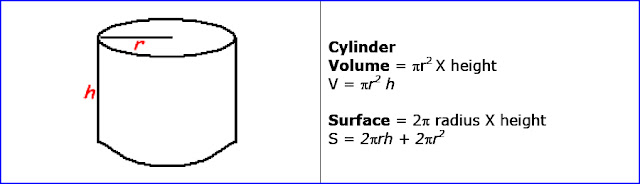# C++ Exercises

## C++ Program to calculate the volume and surface of the cylinder.

Let see the program to calculate the volume and surface of the cylinder.

```
Example:

Formula:Cylinder

```
```
/* to calculate the volume of the cylinder. */
#include <iostream>

using namespace std;
int main()
{

cout<<"Enter height of cylinder :";
cin>>height;
cout<<"Volume of cylinder = "<<volume<<" units."<<endl;

return 0;
}
```
##### Output:
```
Enter height of cylinder :9.3

Volume of cylinder = 906.471 units.

```
```
/* to calculate the surface of the cylinder. */
#include <iostream>

using namespace std;
int main()
{

cout<<"Enter height of cylinder :";
cin>>height;
cout<<"surface of cylinder = "<<volume<<" sq. units."<<endl;

return 0;
}

```
```